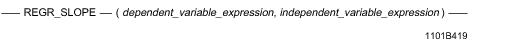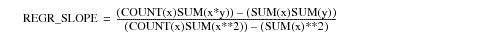# 15.00 - REGR_SLOPE - Teradata Database

## Teradata Database SQL Functions, Operators, Expressions, and Predicates

Product
Release Number
15.00
Content Type
Programming Reference
Publication ID
B035-1145-015K
Language
English (United States)

## REGR_SLOPE

### Purpose

Returns the slope of the univariate linear regression line through all non-null data pairs of the dependent and independent variable arguments.

### Syntaxwhere:

 Syntax element … Specifies … dependent_variable_expression the dependent variable for the regression. The expression cannot contain any ordered analytical or aggregate functions. independent_variable_expression the independent variable for the regression. The expression cannot contain any ordered analytical or aggregate functions.

### ANSI Compliance

This is ANSI SQL:2011 compliant.

### Definition

The slope of the best fit linear regression is a measure of the rate of change of the regression of one independent variable on the dependent variable.

The plot of the linear regression on the variables is used to predict the behavior of the dependent variable from the change in the independent variable.

Note that this computation assumes a linear relationship between the variables.

There can be a strong nonlinear relationship between independent and dependent variables, and the computation of the simple linear regression between such variable pairs does not reflect such a relationship.

### Independent and Dependent Variables

An independent variable is a treatment: something that is varied under your control to test the behavior of another variable.

A dependent variable is something that is measured in response to a treatment.

For example, you might want to test the ability of various promotions to enhance sales of a particular item.

In this case, the promotion is the independent variable and the sales of the item made as a result of the individual promotion is the dependent variable.

### Setting Up Axes for Plotting

If you export the data for plotting, define the y-axis (ordinate) as the dependent variable and the x-axis (abscissa) as the independent variable.

### Combination With Other Functions

REGR_SLOPE can be combined with ordered analytical functions in a SELECT list, QUALIFY clause, or ORDER BY clause. For more information on ordered analytical functions, see Chapter 22: “Ordered Analytical / Window Aggregate Functions.”

REGR_SLOPE cannot be combined with aggregate functions within the same SELECT list, QUALIFY clause, or ORDER BY clause.

### Computation

The equation for computing REGR_SLOPE is defined as follows:where:

 This variable … Represents … x independent_variable_expression y dependent_variable_expression

When there are fewer than two non-null data point pairs in the data used for the computation, then REGR_SLOPE returns NULL.

Division by zero results in NULL rather than an error.

### Result Type and Attributes

The data type, format, and title for REGR_SLOPE(y, x) are as follows.

 Data Type Format Title REAL Default format of the REAL data type REGR_SLOPE(y,x)

For information on the default format of data types and the formatting characters in the format, see “Data Type Formats and Format Phrases” in SQL Data Types and Literals.

### Support for UDTs

By default, Teradata Database performs implicit type conversion on UDT arguments that have implicit casts that cast between the UDTs and any of the following predefined types:

• Numeric
• Character
• DATE
• Interval
• To define an implicit cast for a UDT, use the CREATE CAST statement and specify the AS ASSIGNMENT clause. For more information on CREATE CAST, see SQL Data Definition Language.

Implicit type conversion of UDTs for system operators and functions, including REGR_SLOPE, is a Teradata extension to the ANSI SQL standard. To disable this extension, set the DisableUDTImplCastForSysFuncOp field of the DBS Control Record to TRUE. For details, see Utilities: Volume 1 (A-K).

For more information on implicit type conversion of UDTs, see Chapter 13: “Data Type Conversions.”

### REGR_SLOPE Window Function

For the REGR_SLOPE window function that performs a group, cumulative, or moving computation, see “Window Aggregate Functions” on page 984.

### Example

This example uses the data from the HomeSales table.

 SalesPrice NbrSold Area 160000 126 358711030 180000 103 358711030 200000 82 358711030 220000 75 358711030 240000 82 358711030 260000 40 358711030 280000 20 358711030

The following query returns the slope of the regression line for NbrSold and SalesPrice in the range of 160000 to 280000 in the 358711030 area.

`   SELECT CAST (REGR_SLOPE(NbrSold,SalesPrice) AS FLOAT)  `
`   FROM HomeSales`
`   WHERE area = 358711030 `
`   AND SalesPrice BETWEEN 160000 AND 280000;`

Here is the result:

`   REGR_SLOPE(NbrSold,SalesPrice)`
`   ------------------------------`
`           -7.92857142857143E-004`